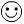# Solving Inequalities

Solving inequalities uses the same rules and solving an equation. The only additional rule will be is if you multiply or divide by a negative number you have to reverse the inequality symbol.

I. One Step Inequalities

1. x + 4 < 5 2. x - 5 ≥ -10 3. 4x > 20 4. (2) $\frac{x}{2}$(2)
-4   -4+5   +5  4    4
x < 1 x ≥ -5 x > 4 x ≤ 24

5. -3x15 6. (-2) $\frac{x}{-2}$ < 5 (-2) flip the inequality
-3    -3 flip the inequality
x ≤ -5 x > -10

A note of caution7. 2x > -12 Do you flip???? NO The number you would divide by is positive
22

8. (4)$\frac{x}{4}$ ≥ -8 (4) Do you flip??? NO The number you multiply by is positive

II. Two step Inequalities

1. 3x + 4 > 10 2. -4x + 5 ≥ 21
-4   -4 -5    -5
3x > 6 -4x16
3     3  -4     -4 dividing by a negative

x > 2 x ≤ -4 FLIP the inequality symbol

Challenge 2 > 6 - x   flip entire inequality so x is on the left

6 - x < 2    now solve
-6    -6
- x < -4
-1    -1    dividing by a negative number!!!

x > 4    Flip the inequality symbol

III. Multi-step Inequalities

1. 6(x + 1) > 6

6x + 6 > 6   distributive property

6x > 0   subtract 6 from both sides

X > 0   divide both sides by 6

2. 10 - 11x > -5x -4

10 - 6x > -4   subtract (-5x) from both sides

-6x > -14   subtract 10 from both sides

X < 7/3   divide by (-6) and flip the inequality symbol

IV. Special Cases

1. 7x - 11x + 3 ≥ 3 - 4x

-4x + 3 ≥ 3 - 4x   combine like terms
+4x         +4x  add 4x to both sides
3 ≥ 3 this is a true statement because 3 = 3

The solution is All Real Numbers

2. 4(3-2x) > 2(6 - 4x)

12 - 8x > 12 -8x   distributive property
+8x     +8x   add (8x) to both sides
12 > 12    this is a false statement - 12 is not greater than 12

There is No Solution

Let's wrap it up. You can solve inequalities just like solving an equation. Be cautious when solving inequalities that you make sure to flip the inequality symbol when you multiply or divide by a negative number. If the variable is eliminated from the equation then you will have a special case. If you get a true statement then the solution is "all real numbers". If you get a false statement then the solution is "no solution".

 Related Links: Math algebra Dependent and Independent Variables Measures of Variability : Mean Absolute Deviation

To link to this Solving Inequalities page, copy the following code to your site: